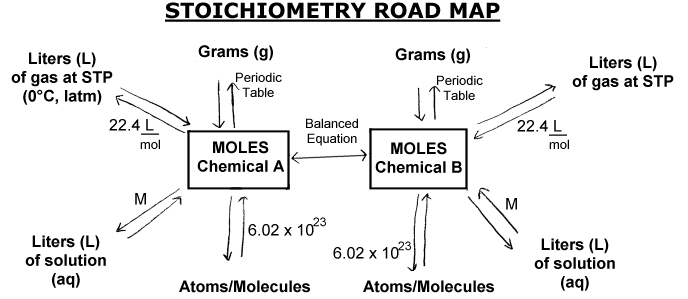Chemistry

# What is stoichiometry?

A chemical reaction is the process in which a substance (or substances) changes to form one or more new substances, i.e. it is a process of change of some reactive initial to final products.
Chemical reactions are represented by chemical equations. For example the carbon (C) could react with oxygen gas (O2) to form carbon dioxide (CO2). The chemical equation for this reaction is written:
C + O2 = CO2
The ‘ +’ is read as “react with” and the arrow means “produces”. To the left of the arrow chemical formulas represent reagents known as starting substances. To the right of the arrow are the formulas chemical of the substances produced as reaction products. The numbers beside the formulas are the coefficients (the coefficient 1 is omitted).
In the previous reaction C and O2 are the reagents, the CO2 product.

The reaction of formation of water is written:

2 H 2 + O2 = 2H2O

note in the previous reaction (formation of the water) that the number of atoms of each element on each side of the equation is the same:

according to the law of conservation of mass atoms are neither created nor destroyed, during a chemical reaction. Therefore a chemical equation must have the same number of atoms of each element on both sides of the arrow. It is said that the equation is balanced.

How do you balance chemical equations?

1) its a standardized procedure described below:

2) determine reagents and chemical reaction products

3) will write the chemical equation reagent to products

4) balancing the equation of the following form:

-begins to match the equation trying different coefficients to ensure that the number of atoms of each element is equal in both sides of the equation. The subscripts in formulas cannot be changed.

-First look for elements that appear only once on each side of the equation and with the same number of atoms: the formulas containing these elements must have the same coefficient. Therefore, it is not necessary to adjust the coefficients of these elements at that time.

-Then look for items that appear only once on each side of the equation, but with different number of atoms and these elements are balanced. The elements that appear in two or more formulas on the same side of the equation are finally balanced.

-Verifies that the igualada equation has the same total number of atoms of each type on both sides of the equation arrow.

Example:
consider the combustion of the gas methane (CH4). This reaction consumes oxygen (O2) and produces carbon dioxide (CO2) and water (H2O). We can then write the chemical equation:

CH4 + O2 = CO2 + H2O

now count the number of atoms of each element in reagents and products:

reagents:
C = 1, H = 4, or = 2

products:
C = 1, H = 2, or = 3

carbon and hydrogen are in a compound of reagents and other products.

There are the same number of carbon atoms in the reagents that products, but twice more hydrogen in reagents that products and 1.5 times more oxygen in the products that the reagents.

This can be arranged by balancing the reaction, in order to match the number of atoms of each chemical species on each side of the equation. The carbon already is matched, or not have to do anything. For the H must be multiplied by two (2) in the water, thus there are now 4 H atoms on each side. But now we have that right (products) there are 4 atoms of oxygen (two of CO2 and two of the 2H2O), while that the only two left, which must be multiplied by two oxygen left (reagents)

CH4 + 2O2 = CO2 + 2H2O

The ideal is that in a chemical reaction reagents were in the correct proportion stoichiometric, i.e. in the proportion that describes the balanced chemical equation. However, usually tends to be to use an excess of one or more reagents, to get as much as possible of the less abundant reagent to react.